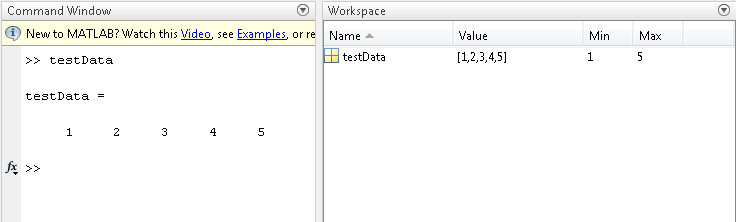Main Content

## Create Diagonal Matrix Using Worksheet Cells

This example shows how to execute Spreadsheet Link™ functions to export a named range in the worksheet to MATLAB® and create a diagonal matrix using Microsoft® Excel® worksheet cells.

The example assumes that MATLAB is running after Microsoft Excel opens. For details, see Start and Stop Spreadsheet Link and MATLAB.

In a worksheet, enter the numbers 1 through 5 into the range of cells from `A1` through `E1`. Define the name `testData` for this range of cells and select it. For instructions, see Excel Help and enter the search term: define and use names in formulas.

The named range `testData` appears in the Name Box.Enter the Spreadsheet Link function `MLPutRanges` directly into the worksheet cell as a worksheet formula. Double-click cell `A3`. Enter this text.

```=MLPutRanges() ```

Press Enter. Microsoft Excel exports the named range `testData` into the MATLAB variable `testData` in the MATLAB workspace. After a Spreadsheet Link function successfully executes as a worksheet formula, the cell contains the value `0`. While the function executes, the cell shows the entered formula.Double-click cell `A5`. Next, create a diagonal matrix. Use the `diag` function to specify `testData` as the input argument and `d` as the output argument. The Spreadsheet Link function `MLEvalString` executes the MATLAB command. Enter this text.

```=MLEvalString("d = diag(testData);") ```

Press Enter. MATLAB executes the `diag` function. The MATLAB variable `d` appears in the MATLAB workspace and contains the diagonal matrix.

Double-click cell `A7`. Now retrieve the diagonal matrix into the worksheet using the Spreadsheet Link function `MLGetMatrix`. Enter this text.

```=MLGetMatrix("d","A9") ```

The diagonal matrix displays in cell `A9` through `E13`.## SupportGet trial now